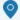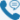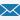#### 新闻中心地 址：市翔安区火炬园二期翔安西路8081号电 话：0592-7270102邮箱：busi2@fj-lide.com

# 弯曲过程及相关过程

* 来源: * 作者: * 发表时间: 2020-01-17 0:11:49 * 浏览: 148
[文字]最小折弯尺寸与V形槽的尺寸有关，与折弯的上模无关。下表为“ ldquo”，常用折弯为“ ld”，之字形折弯a = 90ordm，最小折弯尺寸计算公式和数据，当a> 90ordm或<90ordm时，h值需要相应地增大或减小。材料厚度v型槽尺寸弯曲角度（a） 曲半径（在r内）普通弯曲的最小尺寸（h="0.5V" +="" t）之字形弯曲最小尺寸（h="0.5V" +="" 2t="" +="" 1.5="" +="" r内部）最小距离从孔到弯曲边缘（l="0.6V）T" =="" 0.5v4a="90ordm，/" r="0.62.75.11。" 9t="0.8V4a" =="" 90ordm，/r="0.63.05.72.4T=1.0V6a=90ordm，/R=0.64.07.13.3T=1.2V6a=90ordm，/R=0.64.37.53.6T=1.5V8a=90ordm,/" r="0.65.59.14.8T" =="" 2.0v10a="90ordm，/" r="0.6711.16T" =="" 2.5v12a="90ordm，/" r="0.68.5.17.2T" =="" 3.0v16a="90ordm，/" r="0.61116.19.6">